# 1 SDF

SDF，即signed distance field，有向距离场or带符号距离场（直译）。

## 1.1 记录了什么

SDF贴图，每个像素记录自己与距离自己最近的物体边界的距离。也就是说，SDF的贴图记录的竟然不是颜色信息，而是距离。

### 图像的矢量表达

Signed Distance Field与Multi-channel signed distance field这篇文章更加直白的介绍了SDF——SDF本质上就是在一个光栅图里存了一个图像的矢量表达，说的太好了，真得就是这么一回事。

### signed

1（直接截图了Signed Distance Field的内容）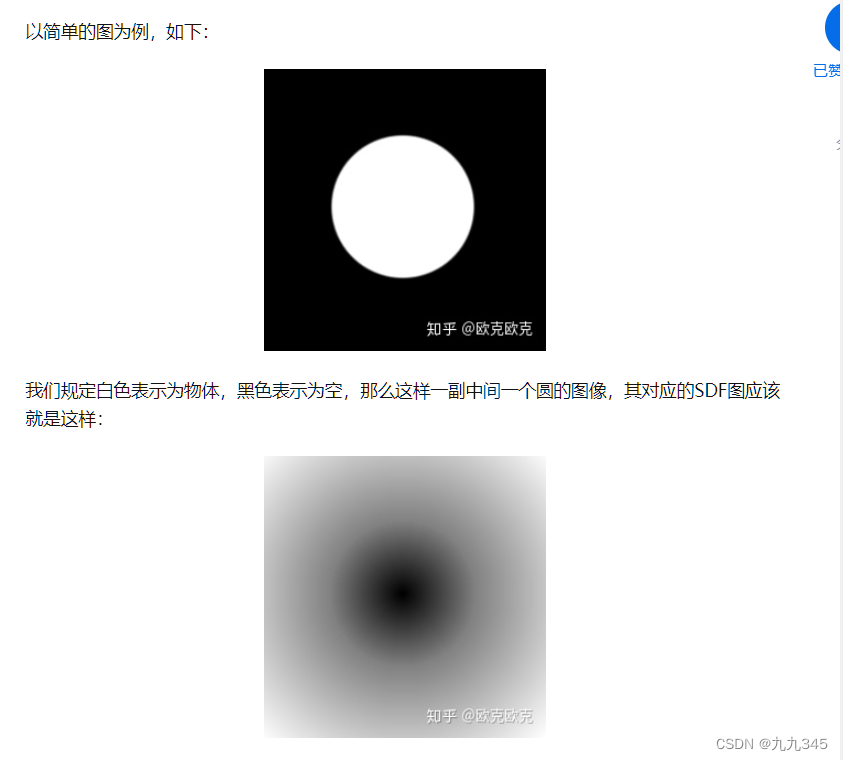2 直接了当的展示了signed（直接截图了SDF(signed distance field)基础理论和计算）：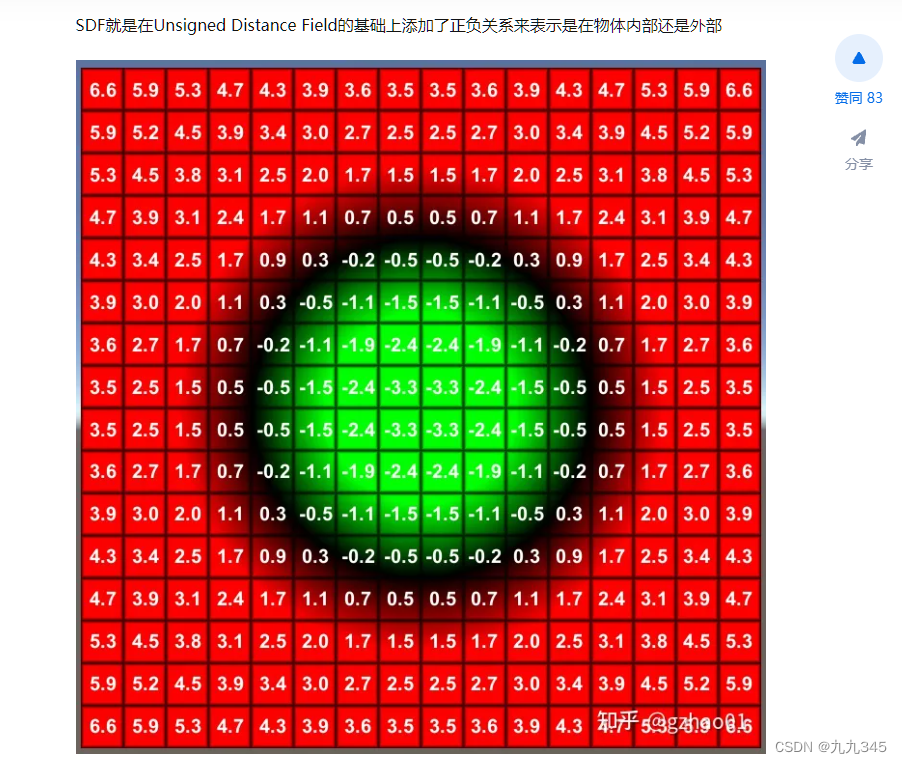3 这个表示方法更加形象理解“矢量”这个描述（直接截图了Signed Distance Field与Multi-channel signed distance field）：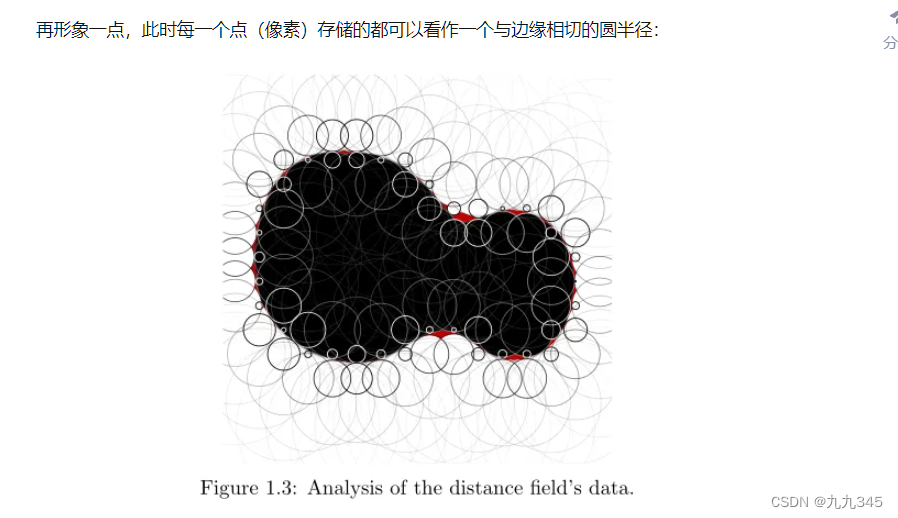## 1.2 能干什么

### Ray Marching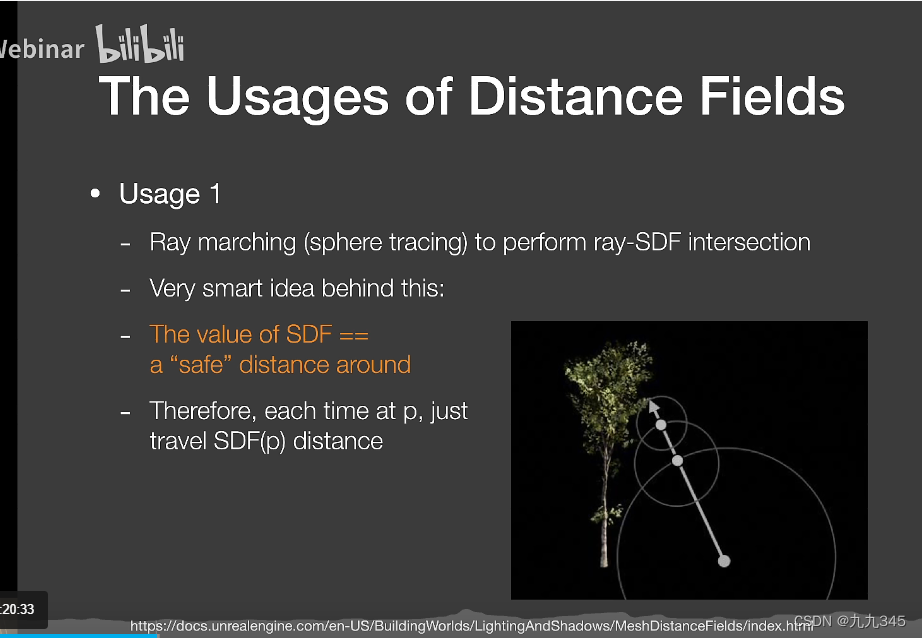### 卡渲的面部光照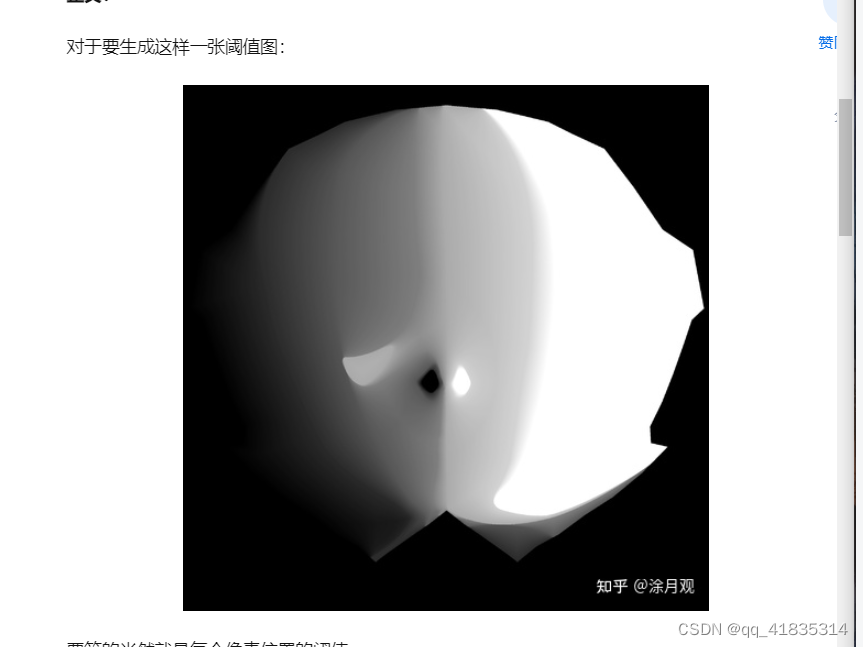SDF可以实现图像之间的平滑过渡，直接对两张SDF进行lerp插值就能得到视觉上的平滑过渡效果实际上是SDF贴图记录的距离之间的平滑过渡。

## 1.3 怎么做

8SSEDT算法（8-points Signed Sequential Euclidean Distance Transform），这是一种能在线性时间内计算出SDF的算法，基本上实现SDF都用的是8SSEDT吧。下面会着重学习一下这个算法是如何实现的。

# 2 8SSEDT算法

8ssedt其实是实现SDF的一种算法，还有其他方法，至于方法之间的对比以及简易程度这里我就略过啦！

## 2.1 算法核心：递推

SDF的宗旨一直都是记录当前像素点离最近物体边界的距离。我们假设用0和1来表示当前像素点，0表示像素点值为空，1表示在物体，那么要找到距离当前点最近的目标像素点，就有以下两种情况：

• 像素值为1：代表像素点自己就是目标点，距离为0
• 像素值为0：代表当前像素点不是目标点，意味着向四周任意方向（上下左右/左上左下右上右下）都有可能是目标点

• 上下左右的某个像素点的像素值为1
• 左上左下右上右下的某个像素点的像素值为1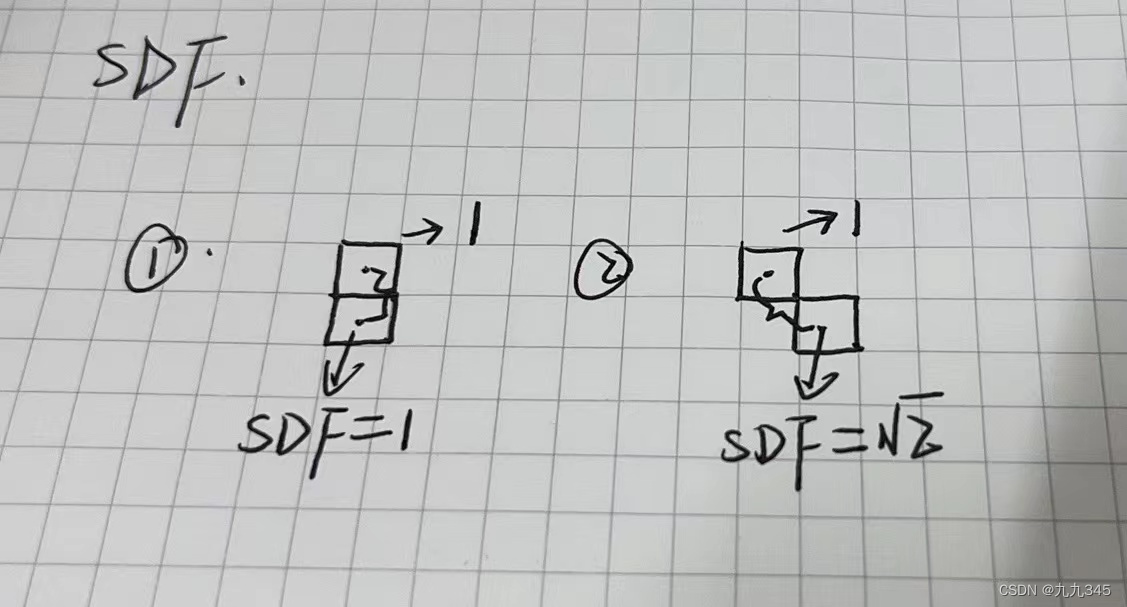### 伪代码

``````now.sdf = 999999;// 初始为空，距离尽可能大
if(now in object){//
now.sdf = 0;// sdf距离为0
}else{// 像素点值为0
foreach(near in nearPixel(now)){
now.sdf = min(now.sdf,near.sdf + distance(now,near));// 递推
}
}
``````

## 2.2 算法核心思路

### STEP1 加载图片并创建2个Grid

• 一个Grid用于计算物体外到物体的距离：那么从距离的角度来讲，我们可以标记白色像素距离为0，黑色像素距离为一个尽可能大的数（例如上面的now.sdf = 999999），这个Grid用于推导向外的距离场
• 一个Grid用于计算物体内部点到物体外的距离：即黑色像素标记距离为0，白色像素标记为一个尽可能大的值，这个Grid用于推导向内的距离场

### STEP3 两个Grid作差

grid1(pixel).sdf - grid2(pixel).sdf就行。

## 2.3 Excel直观理解算法

### STEP1 计算Grid1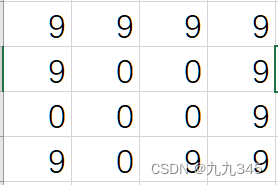PASS0后：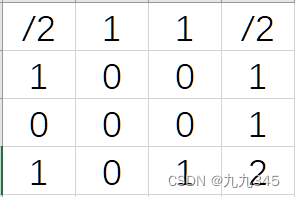PASS1后最终：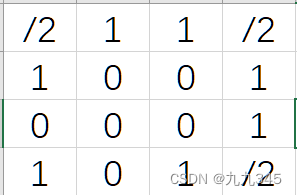### STEP2 计算Grid2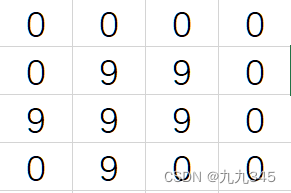PASS0和PASS1后最终：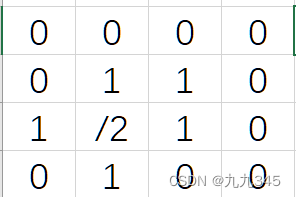### STEP3 相减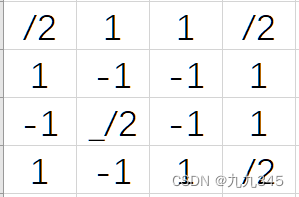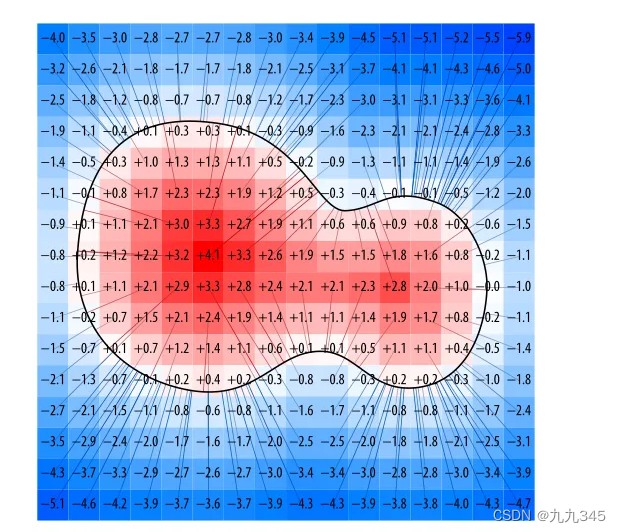# 3 代码实现

## 3.1 SDL库

SDL库的介绍与安装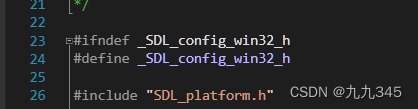## 3.2 最后的完整代码

``````
#include <SDL.h>
#include <SDL_main.h>
#include <math.h>

#pragma comment(lib, "SDL2.lib")
#pragma comment(lib, "SDL2main.lib")

#define SDL_MAIN_HANDLED

#define WIDTH  256
#define HEIGHT 256

struct Point
{
//dx,dy表示对于当前点的偏移值
int dx, dy;

int DistSq() const { return dx * dx + dy * dy; }
};

struct Grid
{
Point grid[HEIGHT][WIDTH];
};

Point inside = { 0, 0 };
Point empty = { 9999, 9999 };
Grid grid1, grid2;

Point Get(Grid& g, int x, int y)
{
// OPTIMIZATION: you can skip the edge check code if you make your grid
// have a 1-pixel gutter.
if (x >= 0 && y >= 0 && x < WIDTH && y < HEIGHT)
return g.grid[y][x];
else
return empty;
}

void Put(Grid& g, int x, int y, const Point& p)
{
g.grid[y][x] = p;
}

void Compare(Grid& g, Point& p, int x, int y, int offsetx, int offsety)
{
//获取当前点偏移后的点
Point other = Get(g, x + offsetx, y + offsety);
//给获取的点的dx和dy设置对应的偏移值
other.dx += offsetx;
other.dy += offsety;

if (other.DistSq() < p.DistSq())
p = other;
}

// Now all we have to do is run the propagation algorithm. See the paper for exactly what's happening here,
// but basically the idea is to see what the neighboring pixel has for it's dx/dy,
//  then try adding it onto ours to see if it's better than what we already have.
void GenerateSDF(Grid& g)
{
// Pass 0
//遍历当前点以及左右、左下、右下、正下方的点，找到距离最短的点存储在网格对应位置处
for (int y = 0; y < HEIGHT; y++)
{
for (int x = 0; x < WIDTH; x++)
{
Point p = Get(g, x, y);
Compare(g, p, x, y, -1, 0);
Compare(g, p, x, y, 0, -1);
Compare(g, p, x, y, -1, -1);
Compare(g, p, x, y, 1, -1);
Put(g, x, y, p);
}

for (int x = WIDTH - 1; x >= 0; x--)
{
Point p = Get(g, x, y);
Compare(g, p, x, y, 1, 0);
Put(g, x, y, p);
}
}

// Pass 1
for (int y = HEIGHT - 1; y >= 0; y--)
{
for (int x = WIDTH - 1; x >= 0; x--)
{
Point p = Get(g, x, y);
Compare(g, p, x, y, 1, 0);
Compare(g, p, x, y, 0, 1);
Compare(g, p, x, y, -1, 1);
Compare(g, p, x, y, 1, 1);
Put(g, x, y, p);
}

for (int x = 0; x < WIDTH; x++)
{
Point p = Get(g, x, y);
Compare(g, p, x, y, -1, 0);
Put(g, x, y, p);
}
}
}

int main(int argc, char* args[])
{
// SDL_Init(SDL_INIT_VIDEO) -- 初始化视频子系统
if (SDL_Init(SDL_INIT_VIDEO) == -1)
return 1;

// 创建一个窗体
SDL_Window* window = SDL_CreateWindow("W", SDL_WINDOWPOS_UNDEFINED, SDL_WINDOWPOS_UNDEFINED, WIDTH, HEIGHT, 0);
// 创建一个与窗体关联的surface，赋值给screen
SDL_Surface* screen = SDL_GetWindowSurface(window);
if (!screen)
return 1;

// 加载位图文件

// 调整窗口
// SDL_ConvertSurface -- 图片被加载时对其以屏幕相同格式进行转化来保证投射过程中不再发生转化，以新的格式返回原来的surface
temp = SDL_ConvertSurface(temp, screen->format, SDL_SWSURFACE);

SDL_LockSurface(temp);
for (int y = 0; y < HEIGHT; y++)
{
for (int x = 0; x < WIDTH; x++)
{
Uint8 r, g, b;
Uint32* src = ((Uint32*)((Uint8*)temp->pixels + y * temp->pitch)) + x;
SDL_GetRGB(*src, temp->format, &r, &g, &b);
// Points inside get marked with a dx/dy of zero.
// Points outside get marked with an infinitely large distance.
// 两个网格，一个内部设置成0，外部设置成正无穷，另一个网格相反。
if (g < 128)
{
Put(grid1, x, y, inside);
Put(grid2, x, y, empty);
}
else {
Put(grid2, x, y, inside);
Put(grid1, x, y, empty);
}
}
}
SDL_UnlockSurface(temp);

// Generate the SDF.
GenerateSDF(grid1);
GenerateSDF(grid2);

// Render out the results.
SDL_LockSurface(screen);
for (int y = 0; y < HEIGHT; y++)
{
for (int x = 0; x < WIDTH; x++)
{
// Calculate the actual distance from the dx/dy
//计算偏移值的点距离当前点的距离
int dist1 = (int)(sqrt((double)Get(grid1, x, y).DistSq()));
int dist2 = (int)(sqrt((double)Get(grid2, x, y).DistSq()));
int dist = dist1 - dist2;

// Clamp and scale it, just for display purposes.
int c = dist * 3 + 128;
if (c < 0) c = 0;
if (c > 255) c = 255;

Uint32* dest = ((Uint32*)((Uint8*)screen->pixels + y * screen->pitch)) + x;
*dest = SDL_MapRGB(screen->format, c, c, c);
}
}
SDL_UnlockSurface(screen);

// Wait for a keypress
SDL_Event event;
while (true)
{
if (SDL_PollEvent(&event))
switch (event.type)
{
case SDL_QUIT:
case SDL_KEYDOWN:
return true;
}
// 更新窗口，才能看到
SDL_UpdateWindowSurface(window);
// 保存成位图
SDL_SaveBMP(screen, "save2.bmp");
}

return 0;
}
``````

## 3.3 运行展示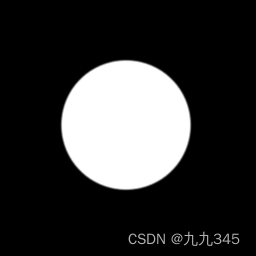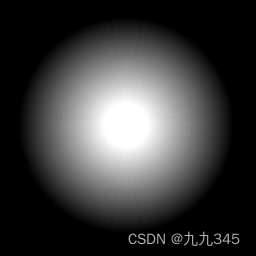PS随便画了一张（需要保存成bmp格式）：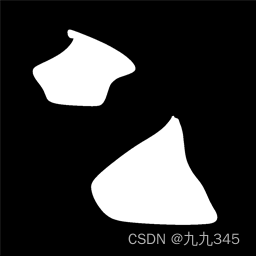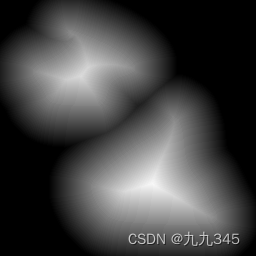# 4 面部阴影(挖个坑)

SDF在风格化渲染中用的最多的地方其实是实现面部阴影，通常是美术绘制好特定光线角度时的面部阴影，通过SDF插值计算出中间的过程，将过程叠加到一张图上，通过简单的blur或者smooth操作实现平滑。

# 参考

（其实参考了非常多的文章，但是后面写着写着忘记了都有哪些了，这里简单的罗列三个吧~）

【有趣的技术】Unity中的SDF(有向距离场) - 简书 (jianshu.com)

Signed Distance Field - 知乎 (zhihu.com)

Tech-Artist 学习笔记：Signed Distance Field 8SSEDT 算法 - 知乎 (zhihu.com)